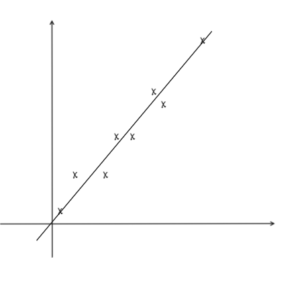# Tag: h2math## Sampling Questions: A Level TYS Answers

Find the worked solutions for past A Level sampling questions, which are found in paper 2 of H2 Math papers, statistics section.## Hypothesis Testing Questions: A Level TYS Answers

Find the worked solutions for past A Level hypothesis testing questions, which are found in paper 2 of H2 Math papers, statistics section.## Regression and Correlation Questions: A Level TYS Answers

Find the worked solutions for past A Level regression and correlation questions, found in paper 2 of H2 Math papers, statistics section.## Binomial Distribution Questions: A Level TYS Answers

Find the worked solutions for past A Level binomial distribution questions. Binomial distribution questions are found in paper 2, statistics section of A Level Math.## Normal Distribution Questions: A Level TYS Answers

Find the worked solutions for past A Level normal distribution questions. Normal distribution questions are found in paper 2, statistics section.## Discrete Random Variable Questions: A Level TYS Answers

Find the worked solutions for past A Level discrete random variable questions, which are found in paper 2 of H2 Math papers, statistics section.## Probability Questions: A Level TYS Answers

Find the worked solutions for past A Level probability questions. Probability questions are found in paper 2.## Permutation and Combination Questions: A Level TYS Answers

Find the worked solutions for past A Level permutation and combination questions. Permutation and combination questions are found in paper 2.## Use of Ti 84 for normal distribution

We talk about the graphic calculator Ti-84 for normal distribution in this post. We’ll look at normal distribution functions in Ti-84: invNorm and normcdf.## Use of Ti 84 for binomial distribution

We talk about the graphic calculator Ti 84 for binomial distribution in this post. We’ll look at 2 binomial distribution functions in Ti 84.